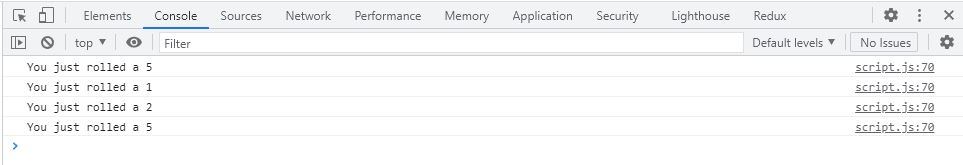# const - JS | lectureJavaScript Fundamentals 2

The while Loop

We learned about the `for loop` in the previous lectures, but there is another type of loop in JavaScript called the `while loop`.

To understand how the `while loop` works and the difference between the `for` and `while loop`, let's consider our very first `for loop` we previously wrote

script.js
``````for (let rep = 1; rep <= 10; rep++) {
console.log(`Weight lifting rep \${rep}`);
}``````

We are going to implement the same thing with the `while loop`. To implement the same thing, we still need the same components .i.e a counter to log the current value to the console, a condition to know when to stop, and we still need to update the counter somehow.

The `while loop` works a bit differently because, in the `while loop`, we can only specify the condition and then manually define the other two components.

script.js
``````let rep = 1;
while (rep <= 10) {
console.log(`Weight lifting rep \${rep}`);
rep++;
}``````

Checking your console, you can see that we get the same result. This means that the `while loop` is more versatile than the `for loop`. Meaning it can be used in a larger variety of situations. That's because it does not really need a counter. We added a counter to our `while loop` because we needed it for this specific use case, but all the `while loop` really needs is a condition that needs to stay true for it to keep running.

The condition can be any condition. It doesn't have to be related to any counter at all, and sometimes that's exactly what we need to solve a certain problem.

For example, let's say we want to keep rolling a dice and stop rolling only when we roll a 6. Essentially we want to keep running our loop while the rolled dice is different from 6. In such a case, we do not know beforehand how many times the loop should run. Meaning we do not need a counter variable.

script.js
``````/**
* Don't worry about the implementation at this point, but just
* to clarify what each method does, let's break it down.
*
* Math.random() * 6 ==> Return a number between 0 and 5 because just Math.random()
* return a number between 0 and 1
*
* Math.trunc ==> Removes de decimal part
*
* The +1 we added is because we need a number between 1 and 6
*/
let dice = Math.trunc(Math.random() * 6) + 1;

while (dice !== 6) {
console.log(`You just rolled a \${dice}`);
dice = Math.trunc(Math.random() * 6) + 1;
}``````

Here is what I have in my console. The result will be different from yours because we have different random numbers.Let's analyze what happened here. We start with some random dice number.

script.js
``let dice = Math.trunc(Math.random() * 6) + 1;``

We then check to see if that dice value is different from 6

script.js
``while (dice !== 6)``

From the above image, we can see that it was actually different. We then entered the loop and logged `You just rolled a 5` and then on the next line, we created a new random number, and the loop ran again

The same thing happened with the other dice values 1, 2 and stopped at 5 because the next dice value was a 6, and so then in the next iteration, dice was equal to 6, making the condition (`dice !== 6`) to be false and the loop to stop.

You might fall in a situation where the first dice value is a 6.

script.js
``let dice = Math.trunc(Math.random() * 6) + 1;``

The loop won't start making the loop have zero iterations. The conclusion is that the `while loop` does not have to depend on any counter variable.

Whenever you need a loop without a counter, you can go for a `while loop`. This happens whenever you do not know beforehand how many iterations the loop will have. Just like the dice, we did not know how many times we would roll a dice that is different from 6. On the other hand, when we know how many times the loop will run, we will need a counter. For example, when we want to loop over an array, we already know how many elements that array has, and so we know how many iterations we will need. Therefore the `for loop` is usually the right device to loop over an array.